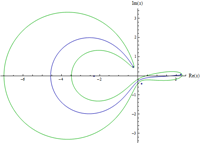February 2011
Mon Tue Wed Thu Fri Sat Sun
« Jan   Mar »
123456
78910111213
14151617181920
21222324252627
28

## Optical Spectral Singularities as Threshold Resonances

Spectral singularities are among generic mathematical features of complex scattering potentials. Physically they correspond to scattering states that behave like zero-width resonances. For a simple optical system, we show that a spectral singularity appears whenever the gain coefficient coincides with its threshold value and other parameters of the system are selected properly. We explore a concrete realization of spectral singularities for a typical semiconductor gain medium and propose a method of constructing a tunable laser that operates at threshold gain.

http://arxiv.org/abs/1102.4695
Optics (physics.optics); High Energy Physics – Theory (hep-th); Mathematical Physics (math-ph); Quantum Physics (quant-ph)

## Periodic orbits for classical particles having complex energy

Alexander G. Anderson, Carl M. Bender, Uriel I. MoroneThis paper revisits earlier work on complex classical mechanics in which it was argued that when the energy of a classical particle in an analytic potential is real, the particle trajectories are closed and periodic, but that when the energy is complex, the classical trajectories are open. Here it is shown that there is a discrete set of eigencurves in the complex-energy plane for which the particle trajectories are closed and periodic.

http://arxiv.org/abs/1102.4822
Mathematical Physics (math-ph); High Energy Physics – Theory (hep-th)